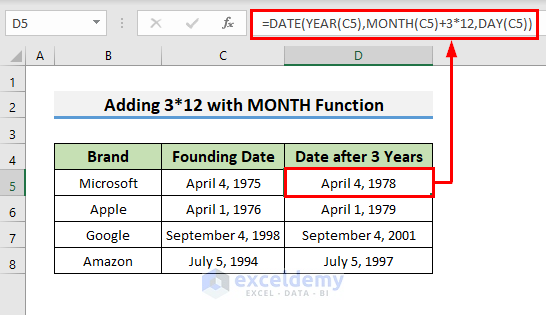# How to Add 3 Years to a Date in Excel (3 Effective Ways)

Get FREE Advanced Excel Exercises with Solutions!

When we work with dates in Excel, sometimes we may want to add specific years to a date. It’s quite easy to do in Excel. At the same time, Excel offers several ways to add years to a date. In this article, I will show you 3 effective ways to add 3 years to a date in Excel. So, without any delay, let’s start the discussion.

We can add 3 years to a date following several methods in Excel. Here, I will discuss 3 effective ways to add 3 years to a date in Excel. For demonstration, I have introduced a dataset having Brand, and Founding Date. We add years in the Date after 3 Years column.## 1. Insert EDATE Function to Add 3 Years to a Date in Excel

The addition of 3 years to a date can be done by using the EDATE function of Excel. I am showing the stepwise procedures for the method in the following.

• First, select Cell D5 and write the following formula there.
`=EDATE(C5,3*12)`
• Then, press Enter.
• Instantly, we will see the date after 3 years in Cell D5.• Further, use the Fill Handle to copy the formula in the cells below.In the formula,

• The EDATE function takes the date from Cell C5 and adds 3*12 months to the date.
• As a consequence, we will see all the dates after years.

## 2. Combine DATE, YEAR, MONTH & DAY Functions Together to Add 3 Years to a Date

We can use the DATE, YEAR, MONTH, and DAY functions altogether to add 3 years to a date. I am showing the stepwise procedure to do that in the below section.

### 2.1. Adding 3 with YEAR Function

We can add 3 to the first argument of the DATE function which is the year. Follow the steps given below for the procedures.

• First, write the following formula in Cell D5.
`=DATE(YEAR(C5)+3,MONTH(C5),DAY(C5))`
• After that, hit Enter.
• Finally, use the Fill Handle to copy the formula in the cells below.
• Instantly, we will see 3 years added to all the dates.In the formula,

• The YEAR function takes the year value from the date of Cell C5 and we added 3 with it.
• The MONTH and DAY functions get the month and day value from the date of Cell C5.
• The DATE function takes the arguments and gives the output date.

### 2.2. Adding 3*12 with MONTH Function

Instead of adding 3 with the YEAR function, we can add 3*12 with the MONTH function to get the same result. Let’s walk through the procedures.

• First, write the following formula in Cell D5.
`=DATE(YEAR(C5),MONTH(C5)+3*12,DAY(C5))`
• Next, press Enter.
• Further, use the Fill Handle to copy the formula in the cells below.
• Simultaneously, we will see all the dates after years.

####In the formula,

• The MONTH function takes the month value from the date of Cell C5 and we added 3*12 with it.
• The YEAR and DAY functions get the year and day value from the date of Cell C5.
• The DATE function takes the arguments and gives the output date.
Note: When the month value is more than 12 the DATE function cuts the value as a multiple of 12 and a year for every 12 months.

Read More: How to Add 2 Years to a Date in Excel

## 3. Apply a Simple Arithmetic Formula to Add 3 Years to a Date in Excel

In addition to the previous methods, we can use a more simplified formula to add 3 years to a date. I am discussing the stepwise procedures below.

• Firstly, write the following formula in Cell D5.
`=C5+3*365`
• Then, hit Enter.
• Afterward, use the Fill Handle to copy the formula in the following cells.
• Finally, we will see the dates after 3 years in each cell.In the formula,

• We have added 3*365 days with the date from Cell C5 to get the date after years.
Note: The formula doesn’t give the exact date after 3 years because we added 365 days for each year but the leap year consists of 366 days. We didn’t account for it in the formula.

## How to Add 3 Months to a Date in Excel

How to add 3 months to a date is quite similar to the addition of 3 years to a date. We can easily do that using the EDATE function of Excel. Let’s follow the stepwise procedures.

• Firstly, write the following formula in Cell D5.
`=EDATE(C5,3)`
• Next, hit Enter.
• Also, use the Fill Handle to copy the formula in the following cells.
• Finally, we will see the dates after 3 years in each cell.In the formula,

• We have used the EDATE function which adds 3 months (as 3 is in the argument) to the date from Cell C5.

Read More: How to Add 6 Months to a Date in Excel

## Conclusion

Adding 3 years to a date is quite a simple task in Excel. Here, I have discussed 3 effective ways to add 3 years to a date in Excel. Please, leave a comment if you have any queries or suggestions.

## What is ExcelDemy?

ExcelDemy Learn Excel & Excel Solutions Center provides free Excel tutorials, free support , online Excel training and Excel consultancy services for Excel professionals and businesses. Feel free to contact us with your Excel problems.Mehedi Hasan Shimul

Hi! I am Mehedi Hasan Shimul. As I am an Engineer solving different problems with the help of Excel amuses me. I write Excel related different problem solving articles here. Hope it will help you.

We will be happy to hear your thoughtsAdvanced Excel Exercises with Solutions PDF